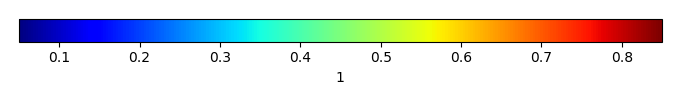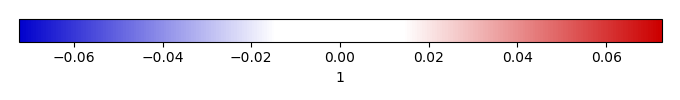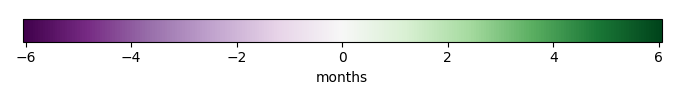# Mean State

Period Mean (original grids) 
Model Period Mean (intersection) 
Model Period Mean (complement) 
Benchmark Period Mean (intersection) 
Benchmark Period Mean (complement) 
Bias 
RMSE 
Phase Shift [months]
Bias Score 
RMSE Score 
Seasonal Cycle Score 
Spatial Distribution Score 
Interannual Variability Score 
Overall Score 
Benchmark [-] 0.175
CLM5PHSOFF [-] 0.158 0.157 0.181 0.0670 -0.0245 0.0514 3.84 0.277 0.328 0.327 0.986 0.483 0.455
CLM5PHSON [-] 0.155 0.154 0.181 0.0670 -0.0265 0.0522 3.97 0.263 0.334 0.311 0.986 0.475 0.450
Period Mean (original grids) 
Model Period Mean (intersection) 
Model Period Mean (complement) 
Benchmark Period Mean (intersection) 
Benchmark Period Mean (complement) 
Bias 
RMSE 
Phase Shift [months]
Bias Score 
RMSE Score 
Seasonal Cycle Score 
Spatial Distribution Score 
Interannual Variability Score 
Overall Score 
Benchmark [-] 0.215
CLM5PHSOFF [-] 0.190 0.192 0.217 0.0596 -0.0250 0.0553 4.20 0.362 0.288 0.270 0.989 0.505 0.450
CLM5PHSON [-] 0.186 0.188 0.217 0.0596 -0.0293 0.0559 3.74 0.327 0.305 0.356 0.991 0.489 0.462
Period Mean (original grids) 
Model Period Mean (intersection) 
Model Period Mean (complement) 
Benchmark Period Mean (intersection) 
Benchmark Period Mean (complement) 
Bias 
RMSE 
Phase Shift [months]
Bias Score 
RMSE Score 
Seasonal Cycle Score 
Spatial Distribution Score 
Interannual Variability Score 
Overall Score 
Benchmark [-] 0.168
CLM5PHSOFF [-] 0.158 0.158 0.173 0.0703 -0.0151 0.0525 4.21 0.301 0.266 0.261 0.901 0.561 0.426
CLM5PHSON [-] 0.157 0.157 0.173 0.0703 -0.0161 0.0509 3.99 0.294 0.292 0.304 0.880 0.529 0.432
Period Mean (original grids) 
Model Period Mean (intersection) 
Model Period Mean (complement) 
Benchmark Period Mean (intersection) 
Benchmark Period Mean (complement) 
Bias 
RMSE 
Phase Shift [months]
Bias Score 
RMSE Score 
Seasonal Cycle Score 
Spatial Distribution Score 
Interannual Variability Score 
Overall Score 
Benchmark [-] 0.281
CLM5PHSOFF [-] 0.356 0.356 0.281 0.273 0.0737 0.224 1.85 0.604 0.305 0.772 0.956 0.792 0.622
CLM5PHSON [-] 0.331 0.330 0.281 0.273 0.0483 0.189 1.54 0.690 0.344 0.828 0.958 0.776 0.657
Period Mean (original grids) 
Model Period Mean (intersection) 
Model Period Mean (complement) 
Benchmark Period Mean (intersection) 
Benchmark Period Mean (complement) 
Bias 
RMSE 
Phase Shift [months]
Bias Score 
RMSE Score 
Seasonal Cycle Score 
Spatial Distribution Score 
Interannual Variability Score 
Overall Score 
Benchmark [-] 0.113
CLM5PHSOFF [-] 0.138 0.138 0.126 0.0694 0.0115 0.0540 3.16 0.355 0.353 0.472 0.0300 0.430 0.332
CLM5PHSON [-] 0.136 0.136 0.126 0.0694 0.00949 0.0536 3.13 0.345 0.360 0.479 0.0178 0.396 0.326
Period Mean (original grids) 
Model Period Mean (intersection) 
Model Period Mean (complement) 
Benchmark Period Mean (intersection) 
Benchmark Period Mean (complement) 
Bias 
RMSE 
Phase Shift [months]
Bias Score 
RMSE Score 
Seasonal Cycle Score 
Spatial Distribution Score 
Interannual Variability Score 
Overall Score 
Benchmark [-] 0.170
CLM5PHSOFF [-] 0.235 0.234 0.170 0.125 0.0636 0.222 1.64 0.477 0.143 0.797 0.654 0.700 0.485
CLM5PHSON [-] 0.201 0.200 0.170 0.125 0.0295 0.145 1.53 0.643 0.226 0.815 0.731 0.704 0.558
Period Mean (original grids) 
Model Period Mean (intersection) 
Model Period Mean (complement) 
Benchmark Period Mean (intersection) 
Benchmark Period Mean (complement) 
Bias 
RMSE 
Phase Shift [months]
Bias Score 
RMSE Score 
Seasonal Cycle Score 
Spatial Distribution Score 
Interannual Variability Score 
Overall Score 
Benchmark [-] 0.176
CLM5PHSOFF [-] 0.206 0.206 0.179 0.0902 0.0274 0.0910 1.49 0.636 0.363 0.774 0.963 0.659 0.626
CLM5PHSON [-] 0.202 0.203 0.179 0.0902 0.0239 0.0841 1.49 0.654 0.381 0.777 0.963 0.653 0.635
Period Mean (original grids) 
Model Period Mean (intersection) 
Model Period Mean (complement) 
Benchmark Period Mean (intersection) 
Benchmark Period Mean (complement) 
Bias 
RMSE 
Phase Shift [months]
Bias Score 
RMSE Score 
Seasonal Cycle Score 
Spatial Distribution Score 
Interannual Variability Score 
Overall Score 
Benchmark [-] 0.142
CLM5PHSOFF [-] 0.160 0.160 0.148 0.0636 0.0116 0.0570 3.98 0.545 0.332 0.316 0.972 0.469 0.494
CLM5PHSON [-] 0.157 0.158 0.148 0.0636 0.00953 0.0556 3.96 0.542 0.343 0.313 0.967 0.442 0.492
Period Mean (original grids) 
Model Period Mean (intersection) 
Model Period Mean (complement) 
Benchmark Period Mean (intersection) 
Benchmark Period Mean (complement) 
Bias 
RMSE 
Phase Shift [months]
Bias Score 
RMSE Score 
Seasonal Cycle Score 
Spatial Distribution Score 
Interannual Variability Score 
Overall Score 
Benchmark [-] 0.307
CLM5PHSOFF [-] 0.356 0.353 0.306 0.330 0.0471 0.187 1.98 0.664 0.369 0.740 0.983 0.765 0.648
CLM5PHSON [-] 0.347 0.345 0.306 0.330 0.0384 0.172 1.85 0.695 0.388 0.768 0.983 0.760 0.664
Period Mean (original grids) 
Model Period Mean (intersection) 
Model Period Mean (complement) 
Benchmark Period Mean (intersection) 
Benchmark Period Mean (complement) 
Bias 
RMSE 
Phase Shift [months]
Bias Score 
RMSE Score 
Seasonal Cycle Score 
Spatial Distribution Score 
Interannual Variability Score 
Overall Score 
Benchmark [-] 0.152
CLM5PHSOFF [-] 0.171 0.171 0.159 0.0651 0.0125 0.0471 2.80 0.457 0.322 0.545 0.843 0.569 0.510
CLM5PHSON [-] 0.168 0.168 0.159 0.0651 0.00995 0.0467 2.64 0.444 0.324 0.576 0.823 0.559 0.508
Period Mean (original grids) 
Model Period Mean (intersection) 
Model Period Mean (complement) 
Benchmark Period Mean (intersection) 
Benchmark Period Mean (complement) 
Bias 
RMSE 
Phase Shift [months]
Bias Score 
RMSE Score 
Seasonal Cycle Score 
Spatial Distribution Score 
Interannual Variability Score 
Overall Score 
Benchmark [-] 0.146
CLM5PHSOFF [-] 0.170 0.170 0.154 0.0654 0.0169 0.0878 2.02 0.598 0.300 0.688 0.976 0.648 0.585
CLM5PHSON [-] 0.166 0.167 0.154 0.0654 0.0131 0.0829 1.98 0.599 0.315 0.697 0.975 0.656 0.593
Period Mean (original grids) 
Model Period Mean (intersection) 
Model Period Mean (complement) 
Benchmark Period Mean (intersection) 
Benchmark Period Mean (complement) 
Bias 
RMSE 
Phase Shift [months]
Bias Score 
RMSE Score 
Seasonal Cycle Score 
Spatial Distribution Score 
Interannual Variability Score 
Overall Score 
Benchmark [-] 0.135
CLM5PHSOFF [-] 0.275 0.275 0.240 0.0836 0.0356 0.100 2.44 0.481 0.327 0.608 0.976 0.571 0.548
CLM5PHSON [-] 0.269 0.269 0.240 0.0836 0.0298 0.0901 2.41 0.501 0.347 0.613 0.973 0.560 0.557
Period Mean (original grids) 
Model Period Mean (intersection) 
Model Period Mean (complement) 
Benchmark Period Mean (intersection) 
Benchmark Period Mean (complement) 
Bias 
RMSE 
Phase Shift [months]
Bias Score 
RMSE Score 
Seasonal Cycle Score 
Spatial Distribution Score 
Interannual Variability Score 
Overall Score 
Benchmark [-] 0.263
CLM5PHSOFF [-] 0.314 0.314 0.271 0.0684 0.0425 0.0749 1.57 0.346 0.361 0.798 0.987 0.522 0.562
CLM5PHSON [-] 0.313 0.313 0.271 0.0684 0.0416 0.0747 1.65 0.346 0.361 0.780 0.986 0.520 0.559
Period Mean (original grids) 
Model Period Mean (intersection) 
Model Period Mean (complement) 
Benchmark Period Mean (intersection) 
Benchmark Period Mean (complement) 
Bias 
RMSE 
Phase Shift [months]
Bias Score 
RMSE Score 
Seasonal Cycle Score 
Spatial Distribution Score 
Interannual Variability Score 
Overall Score 
Benchmark [-] 0.300
CLM5PHSOFF [-] 0.376 0.376 0.301 0.293 0.0751 0.216 1.85 0.611 0.347 0.759 0.965 0.778 0.635
CLM5PHSON [-] 0.366 0.366 0.301 0.293 0.0650 0.196 1.68 0.646 0.377 0.787 0.943 0.769 0.650
Period Mean (original grids) 
Model Period Mean (intersection) 
Model Period Mean (complement) 
Benchmark Period Mean (intersection) 
Benchmark Period Mean (complement) 
Bias 
RMSE 
Phase Shift [months]
Bias Score 
RMSE Score 
Seasonal Cycle Score 
Spatial Distribution Score 
Interannual Variability Score 
Overall Score 
Benchmark [-] 0.110
CLM5PHSOFF [-] 0.150 0.150 0.120 0.0597 0.0294 0.0509 3.35 0.441 0.318 0.443 0.791 0.531 0.474
CLM5PHSON [-] 0.148 0.148 0.120 0.0597 0.0275 0.0493 3.63 0.456 0.332 0.394 0.765 0.496 0.462
Period Mean (original grids) 
Model Period Mean (intersection) 
Model Period Mean (complement) 
Benchmark Period Mean (intersection) 
Benchmark Period Mean (complement) 
Bias 
RMSE 
Phase Shift [months]
Bias Score 
RMSE Score 
Seasonal Cycle Score 
Spatial Distribution Score 
Interannual Variability Score 
Overall Score 
Benchmark [-] 0.121
CLM5PHSOFF [-] 0.166 0.166 0.124 0.0591 0.0413 0.0840 2.26 0.483 0.331 0.636 0.898 0.639 0.553
CLM5PHSON [-] 0.159 0.159 0.124 0.0591 0.0348 0.0683 2.18 0.531 0.372 0.647 0.963 0.639 0.587
Period Mean (original grids) 
Model Period Mean (intersection) 
Model Period Mean (complement) 
Benchmark Period Mean (intersection) 
Benchmark Period Mean (complement) 
Bias 
RMSE 
Phase Shift [months]
Bias Score 
RMSE Score 
Seasonal Cycle Score 
Spatial Distribution Score 
Interannual Variability Score 
Overall Score 
Benchmark [-] 0.151
CLM5PHSOFF [-] 0.201 0.201 0.154 0.0803 0.0480 0.156 1.11 0.550 0.309 0.885 0.859 0.677 0.598
CLM5PHSON [-] 0.174 0.175 0.154 0.0803 0.0219 0.0997 1.05 0.675 0.425 0.894 0.926 0.647 0.665
Period Mean (original grids) 
Model Period Mean (intersection) 
Model Period Mean (complement) 
Benchmark Period Mean (intersection) 
Benchmark Period Mean (complement) 
Bias 
RMSE 
Phase Shift [months]
Bias Score 
RMSE Score 
Seasonal Cycle Score 
Spatial Distribution Score 
Interannual Variability Score 
Overall Score 
Benchmark [-] 0.159
CLM5PHSOFF [-] 0.185 0.185 0.161 0.0596 0.0237 0.0713 0.940 0.616 0.399 0.855 0.984 0.658 0.652
CLM5PHSON [-] 0.176 0.176 0.161 0.0596 0.0147 0.0589 1.10 0.667 0.436 0.828 0.991 0.685 0.674
Period Mean (original grids) 
Model Period Mean (intersection) 
Model Period Mean (complement) 
Benchmark Period Mean (intersection) 
Benchmark Period Mean (complement) 
Bias 
RMSE 
Phase Shift [months]
Bias Score 
RMSE Score 
Seasonal Cycle Score 
Spatial Distribution Score 
Interannual Variability Score 
Overall Score 
Benchmark [-] 0.133
CLM5PHSOFF [-] 0.137 0.137 0.135 0.0638 0.00176 0.0424 3.57 0.728 0.357 0.387 0.454 0.402 0.448
CLM5PHSON [-] 0.135 0.135 0.135 0.0638 -5.25e-05 0.0419 3.87 0.733 0.361 0.330 0.298 0.387 0.412
Period Mean (original grids) 
Model Period Mean (intersection) 
Model Period Mean (complement) 
Benchmark Period Mean (intersection) 
Benchmark Period Mean (complement) 
Bias 
RMSE 
Phase Shift [months]
Bias Score 
RMSE Score 
Seasonal Cycle Score 
Spatial Distribution Score 
Interannual Variability Score 
Overall Score 
Benchmark [-] 0.139
CLM5PHSOFF [-] 0.152 0.152 0.141 0.0683 0.0110 0.0462 4.58 0.650 0.312 0.193 0.906 0.477 0.475
CLM5PHSON [-] 0.149 0.149 0.141 0.0683 0.00773 0.0441 4.69 0.686 0.321 0.178 0.759 0.453 0.453
Period Mean (original grids) 
Model Period Mean (intersection) 
Model Period Mean (complement) 
Benchmark Period Mean (intersection) 
Benchmark Period Mean (complement) 
Bias 
RMSE 
Phase Shift [months]
Bias Score 
RMSE Score 
Seasonal Cycle Score 
Spatial Distribution Score 
Interannual Variability Score 
Overall Score 
Benchmark [-] 0.133
CLM5PHSOFF [-] 0.157 0.157 0.140 0.0680 0.0162 0.0660 3.25 0.447 0.299 0.463 0.898 0.564 0.495
CLM5PHSON [-] 0.159 0.159 0.140 0.0680 0.0185 0.0624 3.37 0.465 0.314 0.441 0.855 0.540 0.488

# Temporally integrated period mean

BENCHMARK MEANMODEL MEANBIASBIAS SCORERMSERMSE SCOREBENCHMARK INTERANNUAL VARIABILITYMODEL INTERANNUAL VARIABILITYINTERANNUAL VARIABILITY SCOREBENCHMARK MAX MONTHMODEL MAX MONTHDIFFERENCE IN MAX MONTHSEASONAL CYCLE SCORESPATIAL TAYLOR DIAGRAMMODEL COLORS# Spatially integrated regional mean

MODEL COLORSREGIONAL MEANANNUAL CYCLEMONTHLY ANOMALYANNUAL CYCLE# All Models

BenchmarkCLM5PHSOFFCLM5PHSON# Data Information

creation_date: Mon Jun 30 23:00:33 PDT 2014

source_file: This product is generated from monthly 1 degree GEWEX SRB Radiation observations